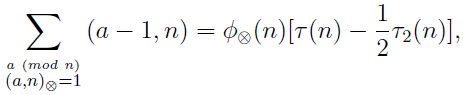# A note on a broken Dirichlet convolution

Emil Daniel Schwab and Barnabás Bede
Notes on Number Theory and Discrete Mathematics, ISSN 1310-5132
Volume 20, 2014, Number 2, Pages 65—73

## Details

### Authors and affiliations

Emil Daniel SchwabDepartment of Mathematical Sciences, The University of Texas at El Paso
El Paso, TX 79968, USA

Barnabás BedeDepartment of Mathematics, DigiPen Institute of Technology
Redmond, WA 98052, USA

### Abstract

The paper deals with a broken Dirichlet convolution ⊗ which is based on using the odd divisors of integers. In addition to presenting characterizations of ⊗-multiplicative functions we also show an analogue of Menon’s identity:where (a, n) denotes the greatest common odd divisor of a and n, φ(n) is the number of integers a (mod n) such that (a, n) = 1, τ(n) is the number of divisors of n, and τ2(n) is the number of even divisors of n.

### Keywords

• Dirichlet convolution
• Möbius function
• Multiplicative arithmetical functions
• Menon’s identity

• 11A25

### References

1. Content, M., F. Lemay, P. Leroux, Catégories de Möbius et fonctorialités: Un cadre général pour l’inversion de Möbius, J. Combin. Theory Ser. A, Vol. 28, 1980, 169–190.
2. Davison, T. M. K. On arithmetic convolutions, Canad. Math. Bull., Vol. 9, 1966, 287–296.
3. Haukkanen, P. On the Davison convolution of arithmetical functions, Canad. Math. Bull., Vol. 32, 1989, 467–473.
4. Haukkanen, P. On a formula for Euler’s totient function, Nieuw Arch. Wisk., Vol. 17, 1999, 343–348.
5. Haukkanen, P. Menon’s identity with respect to a generalized divisibility relation, Aequationes Math., Vol. 70, 2005, 240–246.
6. Kesava Menon, P. On the sum ∑(a − 1, n)[(a, n) = 1], J. Indian Math. Soc., Vol. 29, 1965, 155–163.
7. Lawvere, F. W., M. Menni, The Hopf algebra of Möbius intervals, Theory and Appl. of Categories, Vol. 24, 2010, 221–265.
8. Leinster, T. Notions of Möbius inversion, arXiv: 1201.0413 (2012).
9. Leroux, P. Les catégories de Möbius, Cah. Topol. Géom. Diffé. Catég., Vol. 16, 1975, 280–282.
10. McCarthy, P. J. Introduction to Arithmetical Functions Springer-Verlag, 1986.
11. Schwab, E. D. Complete multiplicativity and complete additivity in Möbius categories,Italian J. Pure and Appl. Math., Vol. 3, 1998, 37–48.
12. Schwab, E. D. Dirichlet convolution, bicyclic semigroup and a breaking process, Int. J. Number Th., Vol. 9, 2013, 1961–1972.

## Cite this paper

APA

Schwab, E. D. & Bede, B. (2014). A note on a broken Dirichlet convolution. Notes on Number Theory and Discrete Mathematics, 20(2), 65-73.

Chicago

Schwab, Emil Daniel, and Barnabás Bede. “A Note on a Broken Dirichlet Convolution.” Notes on Number Theory and Discrete Mathematics 20, no. 2 (2014): 65-73.

MLA

Schwab, Emil Daniel, and Barnabás Bede. “A Note on a Broken Dirichlet Convolution.” Notes on Number Theory and Discrete Mathematics 20.2 (2014): 65-73. Print.